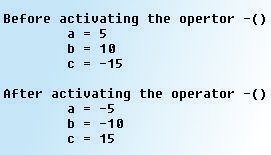•Binary operators can be overloaded in a similar manner as unary operators.

We should note the following features of an operator function for a binary operator:

1. It receives only one class type argument explicitly, in case of a member function. For a friend function, two class types are received as arguments.
2. It returns a class type.

Consider the following expression:

C = A + B;

The above expression contains three objects namely A, B and C of which A and B are the operands while C is the one to which the sum of A and B is assigned to. In the above expression, the operator function is supposed to add two user defined data types and return a user defined data type.Now consider the statement:

temp.x = x + rhs.x;

In the above expression x is the data member of the object that is invoking the operator member function, while rhs.x refers to the data member of the object passed as an argument to the function. Since the function operator + ( ) needs to return the result, we need to have a temp variable (of the same class type) that stores the value to be returned.

It should be noted here that in overloading binary operators the object to the left of the operator is used to invoke the operator function while the operand to the right of the operator is always passed as an argument to the function.

Sample Operator Function

user_defined operator+(user_defined rhs)
{
user_defined temp;
temp.x = x + rhs.x;
temp.y = y + rhs.y;
return temp;
}

We can economize on vertical space by using only the following statement to perform the addition and the return:

return user_defined ((x+B.x),(y+B.y)); // works if appropriate constructor is defined

At this point you must be wondering how we can eliminate a temporary variable and use this statement instead. Well, this statement causes the appropriate constructor to be invoked, and thus initializes an object with no name which stores the result of the addition. This nameless object is then returned by the function. Using temporary objects in this way can make code shorter, more efficient and easier to read.

Sample Program

.cf { font-family: Lucida Console; font-size: 9pt; color:
black; background: white; }
.cl { margin: 0px; }
.cb1 { color: green; }
.cb2 { color: blue; }
.cb3 { color: maroon; }

/*
* Program that illustrates overloading of a binary operator
* http://www.tech-faq.com
*/

#include<iostream>
using namespace std;

class Complex
{
private:
float real, imaginary ;
public:
Complex( )  // Default Constructor without arguments
{ real = 0.0; imaginary = 0.0; }
Complex(float r, float i) // Constructor with arguments
{
real = r ;
imaginary = i ;
}
void enterdata(void)
{
cout << "nEnter the real part of the second Complex number: " ;
cin >> real ;
cout << "nEnter the imaginary part of the second Complex number: " ;
cin >> imaginary ;
}
void display(void)
{
if (imaginary >=0)
cout << real << "+" << imaginary << "i" << endl ;
else
cout << real << imaginary << "i" << endl ;
}
// Operator Function Definition for overloading the binary operator +
Complex operator +(Complex c)
{
Complex temp ;
temp.real = real + c.real ;
temp.imaginary = imaginary + c.imaginary ;
return temp ;
}
// Operator Function Definition for overloading the binary operator -
Complex operator -(Complex c)
{
Complex temp ;
temp.real = real - c.real ;
temp.imaginary = imaginary - c.imaginary ;
return temp ;
}
};  // End of the class definition

int main(void)
{
Complex c1(2.5, 4.5), c2 ;  // Object Definitions
cout << "nThe first Complex number i.e. c1 is: " ;
c1.display( ) ;  // Displays the first Complex number
c2.enterdata( ) ;
cout << "nThe second Complex number i.e. c2 is: " ;
c2.display( ) ;  // Displays the second Complex number
Complex c3 = c1 + c2 ;
cout << "nc1 + c2 is: " ;
c3.display( );   // Displays the third Coplex number
Complex c4 = c1 - c2 ;
cout << "c2 - c1 is: " ;
c4.display( );   // Displays the fourth Complex number
}

Output

The first Complex number i.e. c1 is: 2.5+4.5i

Enter the real part of the second Complex number: 4

Enter the imaginary part of the second Complex number: 5

The second Complex number i.e. c2 is: 4+5i

c1 + c2 is: 6.5+9.5i
c2 - c1 is: -1.5-0.5i

In this Series

176 queries in 0.294 seconds.